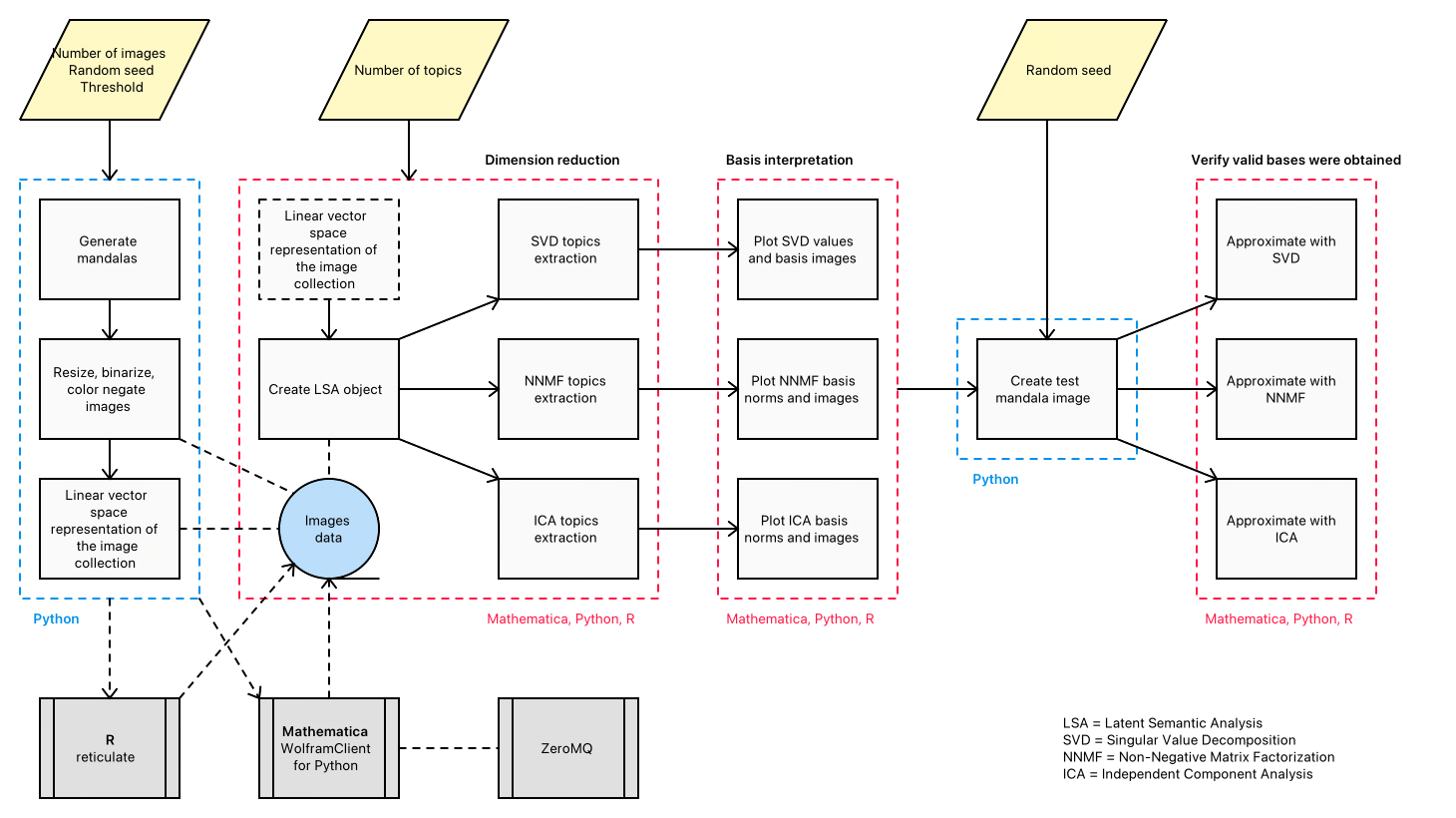# Random mandalas deconstruction in R, Python, and Mathematica

Today (2022-02-28) I gave a presentation Greater Boston useR Meetup titled “Random mandalas deconstruction with R, Python, and Mathematica”. (Link to the video recording.)

Here is the abstract:

In this presentation we discuss the application of different dimension reduction algorithms over collections of random mandalas. We discuss and compare the derived image bases and show how those bases explain the underlying collection structure. The presented techniques and insights (1) are applicable to any collection of images, and (2) can be included in larger, more complicated machine learning workflows. The former is demonstrated with a handwritten digits recognition
application; the latter with the generation of random Bethlehem stars. The (parallel) walk-through of the core demonstration is in all three programming languages: Mathematica, Python, and R.

Here is the related RStudio project: “RandomMandalasDeconstruction”.

Here is a link to the R-computations notebook converted to HTML: “LSA methods comparison in R”.

The Mathematica notebooks are placed in project’s folder “notebooks-WL”.

See the work plan status in the org-mode file “Random-mandalas-deconstruction-presentation-work-plan.org”.

Here is the mind-map for the presentation:The comparison workflow implemented in the notebooks of this project is summarized in the following flow chart:### Articles

[AA1] Anton Antonov, “Comparison of dimension reduction algorithms over mandala images generation”, (2017), MathematicaForPrediction at WordPress.

[AA2] Anton Antonov, “Handwritten digits recognition by matrix factorization”, (2016), MathematicaForPrediction at WordPress.

### Mathematica packages and repository functions

[AAp1] Anton Antonov, Monadic Latent Semantic Analysis Mathematica package, (2017), MathematicaForPrediction at GitHub/antononcube.

[AAf1] Anton Antonov, NonNegativeMatrixFactorization, (2019), Wolfram Function Repository.

[AAf2] Anton Antonov, IndependentComponentAnalysis, (2019), Wolfram Function Repository.

[AAf3] Anton Antonov, RandomMandala, (2019), Wolfram Function Repository.

### Python packages

[AAp2] Anton Antonov, LatentSemanticAnalyzer Python package (2021), PyPI.org.

[AAp3] Anton Antonov, Random Mandala Python package, (2021), PyPI.org.

### R packages

[AAp4] Anton Antonov, Latent Semantic Analysis Monad R package, (2019), R-packages at GitHub/antononcube.

## One thought on “Random mandalas deconstruction in R, Python, and Mathematica”

This site uses Akismet to reduce spam. Learn how your comment data is processed.